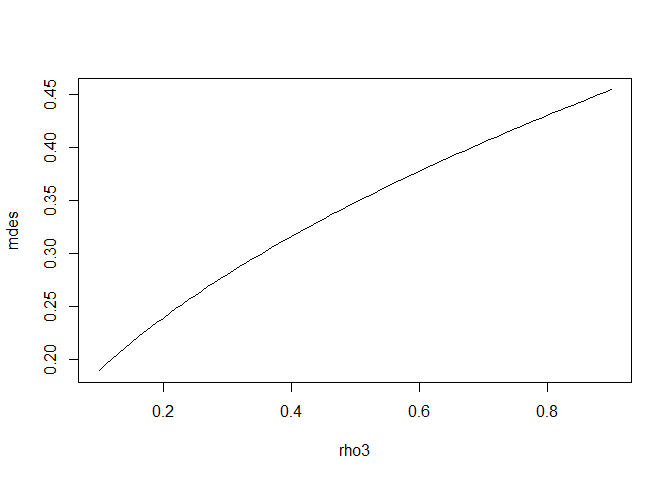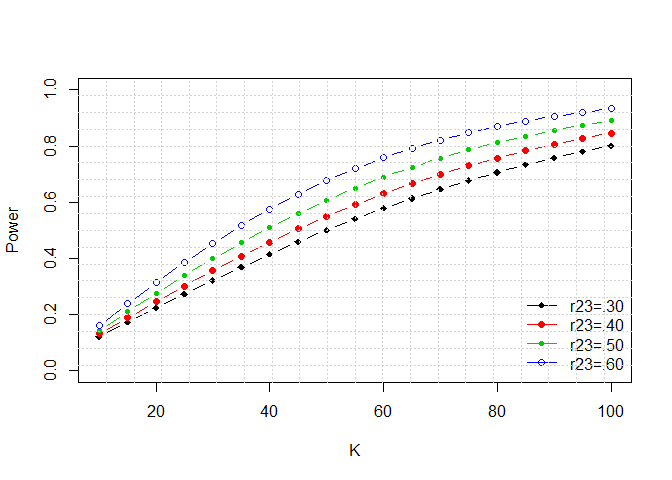# Vectorization of Functions to Create Custom Plots and Tables

#### 2021-10-25

To install and load PowerUpR:

install.packages("PowerUpR")
library(PowerUpR)

PowerUpR functions are not vectorized to avoid possible complications. However, researchers often explore variety of design charactersitics when they determine their sample size. Creating custom plots and tables may assist with their decision. In what follows, example code snippets are provided to demonstrate vectorization of PowerUpR functions over single or multiple design parameters.

NOTE: We would like to thank Dr. Andi Fugard for inspiring this vignette. Dr. Fugard realized that PowerUpR functions does not evaluate arguments when they are embedded within a user-specificed function. This vignette provides example vectorization of PowerUpR functions for creating custom plots and tables.

• Plotting MDES (mdes) against level-3 intra-class correlation coefficient (rho3)
custom_fun <- function(x) {
parms <- list(rho3 = x,
power = .80, rho2 = .06,
g3 = 1, r21 = .55, r22 = .50, r23 = .45,
p = .40, n = 10, J = 2, K = 83)
design <- do.call("mdes.cra3", parms)
design$mdes } x = seq(.10,.90,.01) mdes <- mapply(custom_fun, x) plot(x, mdes, type = "l", xlab = "rho3")• Plotting power (power) against sample size (K) and explanatory power of level-3 covarites (r23) custom_fun <- function(x, y) { parms <- list(K = x, r23 = y, es = .23, rho2 = .06, rho3 = .18, g3 = 1, r21 = .55, r22 = .50, p = .40, n = 10, J = 2) design <- do.call("power.cra3", parms) design$power
}

x = seq(10,100,5)
power.r23.30 <- mapply(custom_fun, x, .30)
power.r23.40 <- mapply(custom_fun, x, .40)
power.r23.50 <- mapply(custom_fun, x, .50)
power.r23.60 <- mapply(custom_fun, x, .60)

# plot
plot(x, power.r23.30, pch = 18, type = "b",
ylim = c(0,1),  xlab = "K", ylab = "Power")
lines(x, power.r23.40, col = 2, pch = 19, type = "b")
lines(x, power.r23.50, col = 3, pch = 20, type = "b")
lines(x, power.r23.60, col = 4, pch = 21, type = "b")
legend("bottomright",  bty = "n",
legend = c("r23=.30", "r23=.40", "r23=.50", "r23=.60"),
col = c(1, 2, 3, 4), lty = c(1, 1, 1, 1), pch = c(18, 19, 20, 21))
grid(nx = 20, ny = 18)• Table of required sample size (K) for various effect size (es) values
custom_fun <- function(x) {
parms <- list(es = x, power = .80, rho2 = .06, rho3 = .18,
g3 = 1, r21 = .55, r22 = .50, r23 = .45,
p = .40, n = 10, J = 2)
design$K } x = seq(.10,.50,.05) K <- mapply(custom_fun, x) table <- data.frame(es = x, K = K) print(table) ## es K ## 1 0.10 431 ## 2 0.15 193 ## 3 0.20 109 ## 4 0.25 71 ## 5 0.30 50 ## 6 0.35 37 ## 7 0.40 29 ## 8 0.45 23 ## 9 0.50 19 • Table of required sample size (K) for various effect size (es) and R-squared values (r23) values custom_fun <- function(x1,x2) { parms <- list(es = x1, r23 = x2, power = .80, rho2 = .06, rho3 = .18, g3 = 1, r21 = .55, r22 = .50, p = .40, n = 10, J = 2) design <- do.call("mrss.cra3", parms) design$K
}

vec.custom_fun <- Vectorize(custom_fun, c("x1", "x2"))

x1 = seq(.10,.50,.05)
x2 = seq(.20,.70,.10)

table.K <- outer(x1, x2, vec.custom_fun)
rownames(table.K) <- paste0("es=",x1)
colnames(table.K) <- paste0("r23=",x2)
print(table.K)
##         r23=0.2 r23=0.3 r23=0.4 r23=0.5 r23=0.6 r23=0.7
## es=0.1      578     519     460     401     342     284
## es=0.15     258     232     206     179     153     127
## es=0.2      146     131     117     102      87      72
## es=0.25      94      85      75      66      57      47
## es=0.3       66      59      53      46      40      33
## es=0.35      49      44      39      35      30      25
## es=0.4       38      34      31      27      23      20
## es=0.45      31      28      25      22      19      16
## es=0.5       25      23      21      18      16      14

–o–